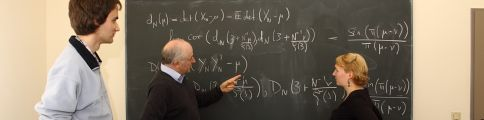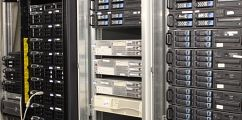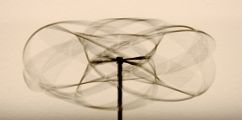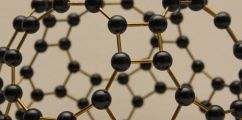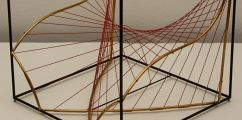# Mathematical programs

This is a list of common used mathematical programs, which are available on our systems. These can be started from terminal.

• GAP
Powerful computer algebra program (for doing algebra on the computer).
• Matlab
Simulation program from MathWorks. Mainly used to solve numerical problems.
• Octave
A free alternative to matlab, which is compatible with main matlab code. qtoctave starts the GUI of octave.
• Maxima
A free computer algebra system. wxmaxima starts the GUI of maxima.
• Maple
A commercial computer algebra system. xmaple starts the GUI of it.
• Geogebra
A program to display dynamic geometry.
• Kile
Frontend for LaTeX.